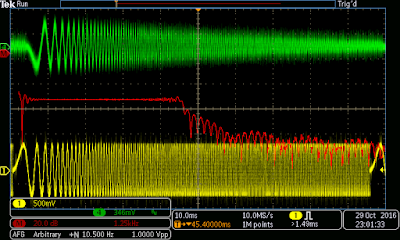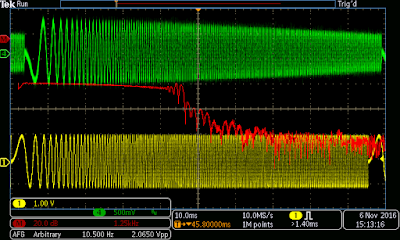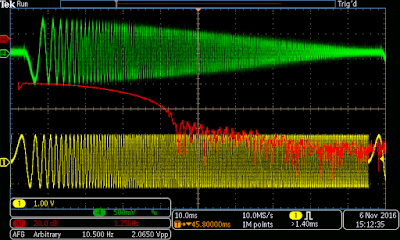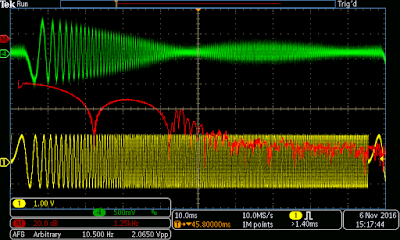## Monday, October 31, 2016

### Moving average Digital Filter implementation TI TMS320 DSP

In post , i am going to show you how to implement the simplest possible digital filter "moving average filter". though it is very easy to implement but still in many applications this is more than good enough. for example reducing random noise from signal. ofcourse when it is very simple it does have issues like , it does no have very sharp filter Response.

Maths

there not much need to be done to calculate output signal. you just need to decide how many point average you want to take for each output point. the more cutoff frequency will go less and less as you increase number of point to be take for average. A 5 point  average  will have pretty high cutoff frequency than a 11 point average.

for a point  a 5 point average is calculated with this simple formula, we take two samples from back and two from front and calculate average for current sample.

$out\left[i\right]=\frac{input\left[i-2\right]+input\left[i-1\right]+input\left[i\right]+input\left[i+1\right]+input\left[i+2\right]}{5}$

little issue come up when i < half of average lenght , because it will make few of the index for input "negative", >as there are very few programming language that support negative index we need to a little adjustment the calculation. we will only take sample from ahead of the current position , this arrangement not going to just going to shift the signal a little ahead in time , other property will remain same.

if you wanted to look at more of  mathematical equation

$out\left[i\right]=\frac{1}{M}\sum _{j=0}^{M-1}input\left[i+j\right]$

M is number of sample taken for averages ,

this whole process is implemented using  convolution operation. if you look at filter kernel for 3 point kernel , it will look like ....0,0,0,1/3,1/3,1/3.0,0,0......

Frequency Response
$out\left[f\right]=\frac{sin\left(\pi fM\right)}{Msin\left(\pi f\right)}$FFT of Input SinalFrequency domain , Pass(1 point 'no avg')5 Point9 Point15 Point19 Point31 Point

Firmware.

Fimrware is quite simple , i use ezdsp5502 board  with on board TMS320VC5502 and TLV320AIC23B codec. TLV320AIC23B codec is used to sample input signal at 48Khz rate and same is used to play back filtered signal , due to limitation you can see the maximum amplitude is limited.
mcBSP is used to transfer sampled data between dsp and TLV320AIC23B,  TLV320AIC23B works as I2S master and send data to DSP , DSP has mcBSP receive interrupt enabled , mcBSP isr receive data and put into a three part ring buffer.

Ring Buffer.
Ring buffer is maintained as three 64 sample long array , one 64 sample long array for input anther for processing and last one for output. these buffer keep on cycle through different stages.

firmware can handle negative array index. though you can easily getaway without this

all source code is available on this github repo.
https://github.com/circuitvalley/TMS320_DSP